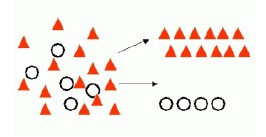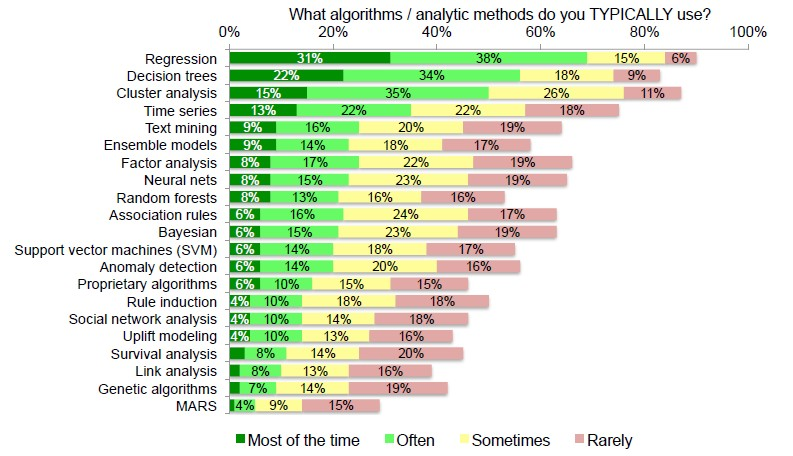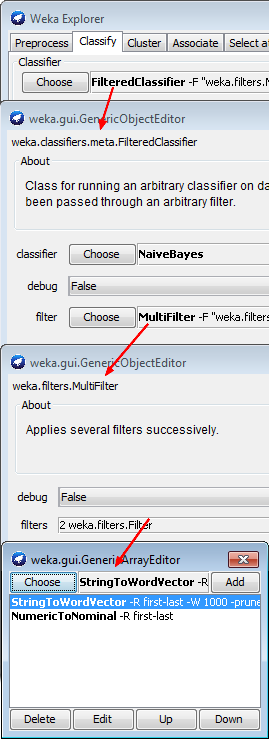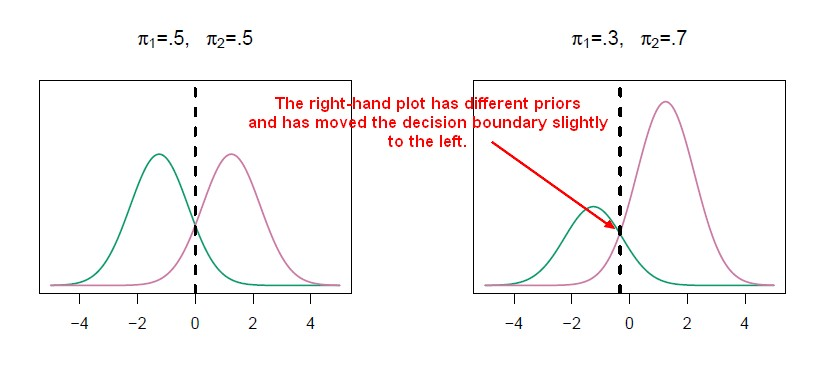# Data Mining - Naive Bayes (NB)

Naive Bayes (NB) is a simple supervised function and is special form of discriminant analysis.

It's a generative model and therefore returns probabilities.

It's the opposite classification strategy of one Rule. All attributes contributes equally and independently to the decision.

Naive Bayes makes predictions using Bayes' Theorem, which derives the probability of a prediction from the underlying evidence, as observed in the data.

Naive Bayes works surprisingly well even if independence assumption is clearly violated because classification doesn’t need accurate probability estimates so long as the greatest probability is assigned to the correct class.

Naive Bayes works also on text categorization.

NB affords fast model building and scoring and can be used for both binary and multi-class classification problems.

The naive Bayes classifier is very useful in high-dimensional problems because multivariate methods like QDA and even LDA will break down.

Naives Bayes is a stable algorithm. A small change in the the training data will not make a big change in the model.

## Assumptions

The fundamental Naive Bayes assumption is that each attribute makes an:

contribution to the outcome.

### Independent

All features are independent given the target Y.

The Naive Bayes assumption say that the probabilities of the different event (ie predictors attributes) are completely independent given the class value. (i.e., knowing the value of one attribute says nothing about the value of another if the class is known)

Independence assumption is never correct but often works well in practice

The attributes must be independent otherwise they will get to much influence on the accuracy. If an attribute are dependent with the target, it's the same that to determine the accuracy of only this attribute.

You can model the dependency of an attribute by copying hem n times. Continuing to make copies of an attribute gives it an increasingly stronger contribution to the decision, until in the extreme the other attributes have no influence at all.

### Equal

The event (ie predictors attributes) are equally important a priori.

If the accuracy remains exactly the same when an attribute is removed, it seems that this attribute is irrelevant to the outcome of the class (target).

## Description

### Formula

The Naive Bayes algorithm is based on conditional probabilities. It uses Bayes' Theorem, a formula that calculates a probability by counting the frequency of values and combinations of values in the historical data.

Bayes' Theorem finds the probability of an event occurring given the probability of another event that has already occurred.

Bayes' theorem: Probability of event A given evidence B

$\text{Prob(A given B)} = \frac\text{Prob(A and B)}}\text{Prob(A)}}$

where:

• P(A) is a priori probability of A (The prior probability) Probability of event before evidence is seen. The evidence is an attribute value of an unknown instance.
• P(A|B) is a posteriori probability of B. Probability of event after evidence is seen. Posteriori = afterwards, after the evidence

### Naive

“Naïve” assumption: Evidence splits into parts that are independent

$P(B|A) = \fracP(A_1|B)P(A_2|B) \dots P(A_n|B)}{P(B)}$ where:

• $A_1, A_2, \dots, A_n$ are totally independent priori.

#### Example

Spam Filtering with Naïve Bayes on a two classes problem (spam and not spam.) Bill Howe, UW

The probability that an email is span given the email words is:

$\begin{array}{rrl} P(spam|words) & = & \fracP(spam) . P(words|spam)}P(words)} \\ & = & \fracP(spam) . P(viagra, rich, \dots, friend|spam)}P(viagra, rich, \dots, friend)} \\ & \propto & P(spam).P(viagra, rich, \dots, friend|spam) \\ \end{array}$

where:

Using the Chain rule:

• One Time: $P(spam|words) \propto P(spam).P(viagra|spam).P(rich, \dots, friend|spam, viagra)$
• Two Time: $P(spam|words) \propto P(spam).P(viagra|spam).P(rich|spam, viagra)*P(\dots, friend|spam, viagra, rich)$

Saying that the word events are completely independent permit to simplify the above formula to a sequential use of the Bayes' formula such as:

$P(spam|words) \propto P(viagra|spam)*P(rich|spam)*\dots*P(friend|spam)$

## Discriminant Function

This is the discriminant function for the kth class for naive Bayes. You compute one of these for each of the classes, then you classify it.

$$\delta_k(x) \approx log \left [ \pi_k \prod^p_{j=1} f_{kj}(x_j) \right ] = -\frac{1}{2} \sum^p_{j=1}\frac{(x_j - \mu_{kj})^2}{\sigma^2_{kj}} + log(\pi_k)$$

There's:

• a determinant term which is a contribution of the feature from the mean for the class scaled by the variance, $\frac(x_j - \mu_{kj})^2}\sigma^2_{kj}$
• a prior term $log(\pi_k)$

NB can be used for mixed feature vectors (qualitative and quantitative).

• For the quantitative ones, we use the Gaussian.
• For the qualitative ones, we replace the density function $f_kj(x_j)$ with probability mass function (histogram) over discrete categories.

And even though it has strong assumptions, it often produces good classification results.

## Example

### Weather Data Set

With the Weather Data Set:

#### P(A and B)

Outlook Temperature Humidity Wind
Yes No P(Yes) P(No) Yes No P(Yes) P(No) Yes No P(Yes) P(No) Yes No P(Yes) P(No)
Sunny 2 3 2/9 3/5 Hot 2 2 2/9 2/5 High 3 4 3/9 4/5 False 6 2 6/9 2/5
Overcast 4 0 4/9 0/5 Mild 4 2 4/9 2/5 Normal 6 1 6/9 1/5 True 3 3 3/9 3/5
Rainy 3 2 3/9 2/5 Cool 3 1 3/9 1/5
Total 9 5 100% 100% Total 9 5 100% 100% Total 9 5 100% 100% Total 9 5 100% 100%

To get rid of the zero (Zero Frequency Problem, 0*n = 0), add one to all the kinds.

#### P(A)

Play P(Yes) / P(No)
Yes 9 9/14
No 5 5/14
Total 14 100%

#### P(A and B)

What is the probability of Yes and No on a new day with the following characteristics (evidence ?): Rainy, Cool, High, True.

$\begin{array}{rrr} P(Yes|New Day) & = & \frac{P(Rainy Outlook |Yes).P(Cool Temperature |Yes).P(High Humidity|Yes).P(With Wind|Yes).P(Play|Yes)}{P(New Day) } \end{array}$

P(New Day) is unknown but it doesn't matter because the same equation can be done for No and it sums up to one with the Yes probability:

$P(yes|New Day)+P(no|New Day)=1$

The proportional probabilty are then:

$\begin{array}{rrrrr} P(Yes|New Day) & \propto & \frac{3}{9} . \frac{3}{9} . \frac{3}{9} . \frac{3}{9} . \frac{9}{14} & \approx & 0.0079 \\ P(No|New Day) & \propto & \frac{2}{5} . \frac{1}{5} . \frac{4}{5} . \frac{3}{5} . \frac{5}{14} & \approx & 0.0137 \\ \end{array}$

This numbers can be converted into a probability by making the sum equal to 1 (normalization):

$\begin{array}{rrrrr} P(Yes|New Day) & = & \frac{0.0079}{0.0079+0.0137} & = & 0.36 \\ P(No|New Day) & = & \frac{0.0137}{0.0079+0.0137} & = & 0.63 \\ \end{array}$

### To test

To calculate the probability of A given B, the algorithm counts the number of cases where A and B occur together and divides it by the number of cases where B occurs alone.

## Documentation / Reference

Discover MoreData Mining - (Classifier|Classification Function)

A classifier is a Supervised function (machine learning tool) where the learned (target) attribute is categorical (“nominal”) in order to classify. It is used after the learning process to classify...Data Mining - Algorithms

An is a mathematical procedure for solving a specific kind of problem. For some data mining functions, you can choose among several algorithms. Algorithm Function Type Description Decision...Data Mining - Generative Model

Generative model Generative models are typically more flexible than discriminative models in expressing dependencies in complex learning tasks. Generative model returns probabilities. Generative model...Data Mining - Maximum Entropy Algorithm

Maximum Entropy (MaxEnt) models are feature-based classifier models. In a two-class scenario, it is the same as using logistic regression to find a distribution over the classes. MaxEnt makes no...Machine learning - Bootstrap aggregating (bagging)

Bootstrap aggregating (bagging) is a machine learning ensemble meta-algorithm designed to improve the stability and accuracy of machine learning algorithms used in statistical classification and regression....Natural Language - Document Classification

document classification aims to classify a document. With where the StringToWordVector filter creates one attribute for each word. Weka...ORE (Oracle R Enterprise)

Oracle R Enterprise is a component of Oracle...R

is a language and environment for statistical computing and graphics. Syntax is very similar to the S_(programming_language)S language. S is a language that was developed by John Chambers et al. at Bell...Statistics - (Confidence|likelihood) (Prediction probabilities|Probability classification)

Prediction probabilities are also known as: confidence (How confident can I be of this prediction?). or likelihood: (How likely is this prediction to be true?) They gives the probability of a predicted...Statistics Learning - Discriminant analysis

Discriminant analysis is a classification method. In discriminant analysis, the idea is to: model the distribution of X in each of the classes separately. use what's known as Bayes theorem to flip...